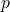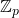# Congruences involving alternating sums related to harmonic numbers and binomial coefficients

Laid Elkhiri, Miloud Mihoubi and Abdellah Derbal
Notes on Number Theory and Discrete Mathematics
Print ISSN 1310–5132, Online ISSN 2367–8275
Volume 26, 2020, Number 4, Pages 39—51
DOI: 10.7546/nntdm.2020.26.4.39-51

## Details

### Authors and affiliations

Laid ElkhiriIbn Khaldoun University, EDPNLHM Laboratory of ENS
Old Kouba, Tiaret, Algeria

Miloud MihoubiUSTHB, Faculty of Mathematics, RECITS Laboratory
PO Box 32 16111 El-Alia, Bab-Ezzouar, Algiers, Algeria

Abdellah DerbalENS, Department of Mathematics, EDPNLHM Laboratory
Old Kouba, Algiers, Algeria

### Abstract

In 2017, Bing He investigated arithmetic properties to obtain various basic congruences modulo a prime for several alternating sums involving harmonic numbers and binomial coefficients. In this paper we study how we can obtain more congruences modulo a power of a prime number(super congruences) in the ring of-integerinvolving binomial coefficients and generalized harmonic numbers.

### Keywords

• Binomial coefficients
• Harmonic numbers
• Generalized harmonic numbers
• Congruences

• 11A07
• 11B65
• 11B99

### References

1. Choi, J., & Srivastava, H. M. (2011). Some summation formulas involving harmonic numbers and generalized harmonic numbers, Mathematical and computer Modelling, 54, 2220–2234.
2. Dilcher, K. (1995). Some q-series identities related to divisor factors, Discrete Math., 145, 83–93.
3. Glaisher, J.W.L. (1900). A general congruence theorem relating to the Bernoullian function, Proc. London Math. Soc., 33, 27–56.
4. Gould, H. W. (1972). Combinatorial Identities. A Standardized Set of Tables Listing 500 Binomial Coefficient Summations, Morgantown, W. Va.
5. He, B. (2017). Some congruences on harmonic numbers and binomial sums, Period. Math. Hung., 74, 67–72.
6. Koparal, S., & Omur, N. (2016). Congruences related to central binomial coefficients, harmonic and Lucas numbers, Turk. J. Math., 40, 973–985.
7. Lehmer, E. (1938). On congruences involving Bernoulli numbers and the quotients of Fermat and Wilson, Ann. Math., 39, 350–360.
8. Mestrovic, R., & Andji, M. (2017). Certain congruence for harmonic numbers, Mathematica Montisnigri, 38.
9. Morley, F. (1895). Note on the congruence 24n ≡ (−1)n(2n)!= (n!)2, where 2n + 1 is a prime, Ann. Math., 9(1–6), 168–170.
10. Prodinger, H. (2000). A q-analogue of a formula of Hernandez obtained by inverting a result of Dilcher, Australas. J. Comb., 21, 271–274.
11. Sun, Z. H. (2000). Congruences concerning Bernoulli numbers and Bernoulli polynomials, Discrete Appl. Math., 105, 193–223.
12. Sun, Z. H. (2008). Congruences involving Bernoulli and Euler numbers, J. Number Theory, 128, 280–312.
13. Sun, Z. W. (2015). Super congruences motivated by e, J. Number Theory, 147, 326–341.
14. Sun, Z. W., & Zhao, L. L. (2013). Arithmetic theory of Harmonic numbers (II), Colloq. Math., 130(1), 67–78.
15. Wang, W. S. (2015). Sums of involving the Harmonic numbers and the Binomial
coefficients, American Journal of Computational Mathematics, 5, 96–105.

## Cite this paper

Elkhiri, L., Mihoubi, M., & Derbal, A. (2020). Congruences involving alternating sums related to harmonic numbers and binomial coefficients, 26(4), 39-51, doi: 10.7546/nntdm.2020.26.4.39-51.